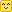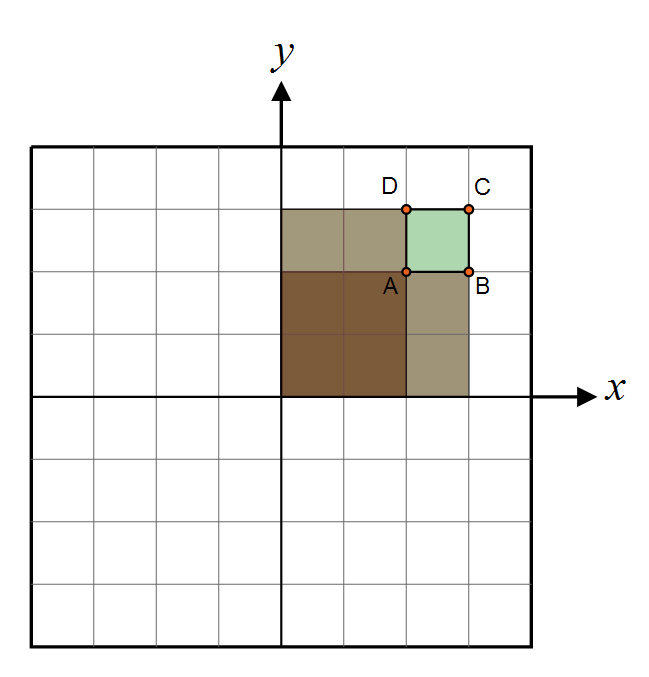Jump to: navigation, search

Hi!

Maybe you already bumped into the problem of projecting a cubemap into spherical harmonics and found this page to help you out: http://www.rorydriscoll.com/2012/01/15/cubemap-texel-solid-angle/ ?

But maybe you need the solution to another, similar problem that consists in finding the solid angle of a pixel lying in the z=0 plane and orthogonally projected onto the hemisphere, but couldn't find a page with that computation?

Well let me help you with that!First of all, the document that was used initially to do the computations -- whether it be in AMD's cube map generator, Rory Driscoll's summary or this page -- is the very interesting thesis by Manne Öhrström.

## Cubemap Projection Configuration

The configuration for a cube map is that pixels are lying on a plane z=1 such as $\mathbf{p'}=(x,y,1), -1<x<1, -1<y<1$ and we project back onto the unit hemisphere by normalizing the vector $\mathbf{p}=\frac{\mathbf{p'}}{|\mathbf{p'}|}=\frac{(x,y,1)}{\sqrt{1+x^2+y^2}}$ as shown on figure 1 above.

The idea is to compute the area of a small element of surface on the hemisphere as we make it vary on the plane, we do that by computing the partial derivatives of $\mathbf{p}$ along x and y that give us the vectors $\frac{\partial \mathbf{p}}{\partial x}$ and $\frac{\partial \mathbf{p}}{\partial y}$.

It is well-known to graphics programmers that computing the cross product of these vectors gives us the normal to the sphere at position $\mathbf{p}$, and the length of that normal is the tiny area element $dA$ on the hemisphere.

Integrating this operation (cross product and norm computation) over an interval $[a,b]\in\mathbb{R}^2$ yields:

$A(x,y) = \int_0^y \int_0^x \left | \frac{\partial \mathbf{p}}{\partial x} \times \frac{\partial \mathbf{p}}{\partial y} \right | \, da \, db \\ = \int_0^y \int_0^x \left (1+a^2+b^2 \right )^{-\frac{3}{2}} \, da \, db \\ = \left | atan( \frac{xy}{\sqrt{1+x^2+y^2}} ) \right | \quad \quad \quad (1)$

It's easy to notice that $A(1,1) = \frac{\pi}{6}$ which is the solid angle covered by a quarter of our cube map face, the solid angle of an entire face would be $\frac{2\pi}{3}$ and all 6 faces of the cube map are then covering a proper solid angle of $4\pi$ and all is well!

Now, for our little problem...

## Our Projection Configuration

Our configuration is a little bit different as our points $\mathbf{p'}(x,y) = (x,y,0)$ and we project onto the hemisphere to obtain $\mathbf{p}(x,y) = (x,y,\sqrt{1-x^2-y^2})$.

The partial derivatives along x and y give:

$\frac{\partial \mathbf{p}}{\partial x} = \begin{pmatrix} 1 \\ 0 \\ -\frac{x}{\sqrt{1-x^2-y^2}} \end{pmatrix}$

$\frac{\partial \mathbf{p}}{\partial y} = \begin{pmatrix} 0 \\ 1 \\ -\frac{y}{\sqrt{1-x^2-y^2}} \end{pmatrix}$

The cross product of these 2 vectors gives:

$\frac{\partial \mathbf{p}}{\partial x} \times \frac{\partial \mathbf{p}}{\partial y} = \frac{1}{\sqrt{1-x^2-y^2}} \begin{pmatrix} x \\ y \\ \sqrt{1-x^2-y^2} \end{pmatrix}$

And the norm is:

$\left | \frac{\partial \mathbf{p}}{\partial x} \times \frac{\partial \mathbf{p}}{\partial y} \right | = \frac{1}{\sqrt{1-x^2-y^2}}$

Which looks kinda nice! Integrating where it is definite (i.e. with x and y in the unit circle) $\int_0^1\int_0^1 \frac{1}{\sqrt{1-x^2-y^2}} dx \, dy = \frac{\pi}{2}$ which is a quarter of the entire hemisphere's solid angle $2\pi$, so we're good!

Except the primitive of this expression is a bit of nightmare after all, but this is our final result:

 $A(x,y) = \int_0^y \int_0^x \frac{1}{\sqrt{1-a^2-b^2}} da \, db = y \tan ^{-1}\left(\frac{x}{\sqrt{-x^2-y^2+1}}\right) + x \tan ^{-1}\left(\frac{y}{\sqrt{-x^2-y^2+1}}\right)+\frac{1}{2} \left(\tan ^{-1}\left(\frac{-x-y^2+1}{y \sqrt{-x^2-y^2+1}}\right)-\tan ^{-1}\left(\frac{x-y^2+1}{y \sqrt{-x^2-y^2+1}}\right)\right) \quad \quad \quad (2)$

I tried to further out the simplification of the last 2 atan terms since a difference of atan gives a single atan but it turns out it shows some precision issues.

## How to use this?

As explained by Driscoll, we compute the area of a single pixel by doing the exact same operation as with Summed Area Tables, that is we compute 4 values for the area of 4 sections like shown here:Then we get: $dA(x,y) = C + A - D - B$

NOTE:

• Obviously, you have to be very careful not to use eq. (2) outside of its region of definition $x^2+y^2 < 1$
• Trying to compute the hemisphere's area using eq. (2) turns out to be converging super slowly: even with a quarter disc split into 10000x10000 pixels, I can only reach 1.5535995614989679...

## Example

Say you have the normalized expression of a Normal Distribution Function D(x,y) defined in an image of the flattened hemisphere:

And you want to compute the shadowing/masking term as given by Dupuy et al eq. 3:

$G(\mathbf{k}) = \frac{cos \theta_k}{\int_{\Omega_+}{\mathbf{k}\mathbf{h} \, D(\mathbf{h}}) \, d\omega_h}$

All you have to do is to write a convolution for each pixel of the image with a code similar to this:

// Perform an integration with the NDF weighted by the cosine of the angle with that particular direction
float3 k = given;
float3 h;
float  convolution = 0.0;
for ( uint Y=0; Y < Height; Y++ ) {
h.y = 2.0f * Y / Height - 1.0f;
for ( uint X=0; X < Width; X++ ) {
h.x = 2.0f * X / Width - 1.0f;
float  sqSinTheta = h.x*h.x + h.y*h.y;
if ( sqSinTheta >= 1.0f )
continue;

h.z = sqrt( 1.0f - sqSinTheta );

float  k_o_h = k.Dot( h );         // k.h
float  D = NDF[X,Y].x;             // D(h)
float  dW = ComputeArea( X, Y );   // dW

convolution += k_o_h * D * dW;
}
}
float	G = k.z / convolution;


This yields the G term as an image that I'm showing here with a strong contrast applied, otherwise it's mostly white: